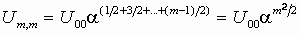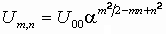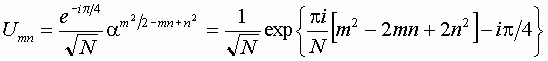Quantization of the Arnold cat map for even values of the quantum parameter

As mentioned, constructing the operator map we face a necessity of selection - to set K'=KA or AK. Both forms are compatible with the classic map but are not equivalent due to non-commutativity of the operators: they differ by factor a, as KA=aAK. Let us consider an "intermediate" variant K'=a1/2KA=a-1/2AK. For the second equation let us keep the symmetric form: A'=AKA.

Substitute expressions for the matrix elements Kmn=dm+1,n and Amn=amdmn into the operator map K'=a1/2KA, A'=AKA and estimate the matrix products. The result is K'mn=dm+1,nan-1/2, A'mn=am+ndm,n-1.

Let us pass now to the Schrodinger representation and find the matrix of the evolution operator U. As we have UK'=KU, we obtain Um,n-1an-1/2=Um+1,n. Analogously, the condition UA'=AU yields Um,n-1a2n-m-1=Umn. As follows from the first expression, the diagonal elements satisfy Um+1,m+1=Ummam+1/2, soNext, with a use of the second expression we have.

It is easy to check that the resulting expression for Umn has the correct period N in respect to both indices. Let us select the initial element as U00=N -1/2e-ip/4. Then, |DetU|=1, and the trace of the matrix appears to be real positive. Finally, we obtainХостинг от uCoz